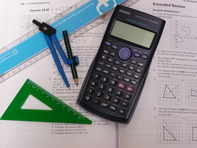# Maths 12-13

£165.00Pack includes: Student file, Textbook, CD for mental maths, and 10 tutor marked assignments including complete SATs-type test.

Covers to level 6:

Numbers and Arithmetic, Approximations, Powers, Multiples, Factors and primes, Fractions and Percentages, Ratio and Proportion, Algebraic Expressions, Equations, Formulas, Sequences, Graphs and Equations, Angles and Shapes, Constructions, Perimeter, Area and Volume and Pythagoras, Transformations, Probability and Statistics.

:  at  £165.00  each